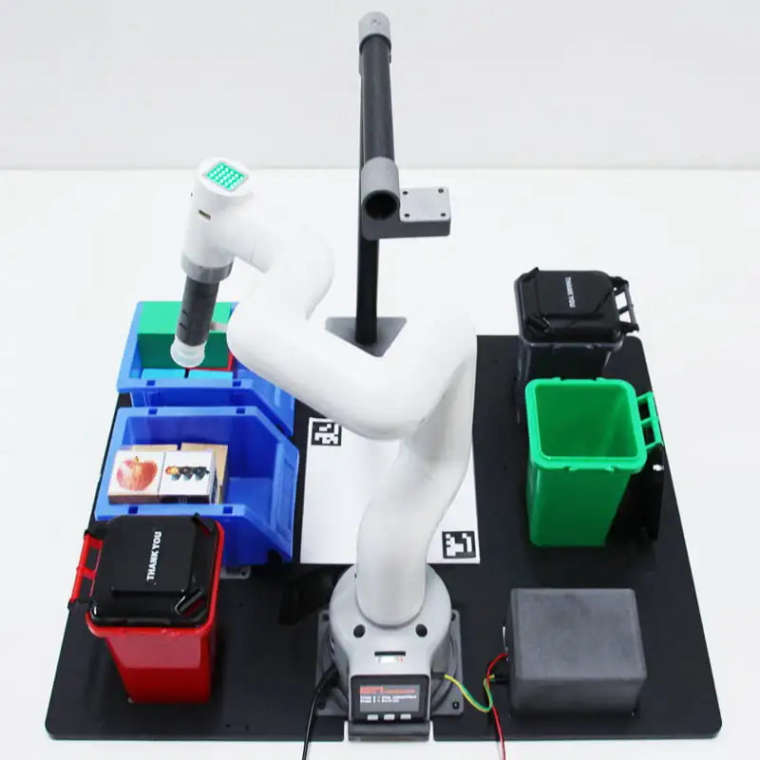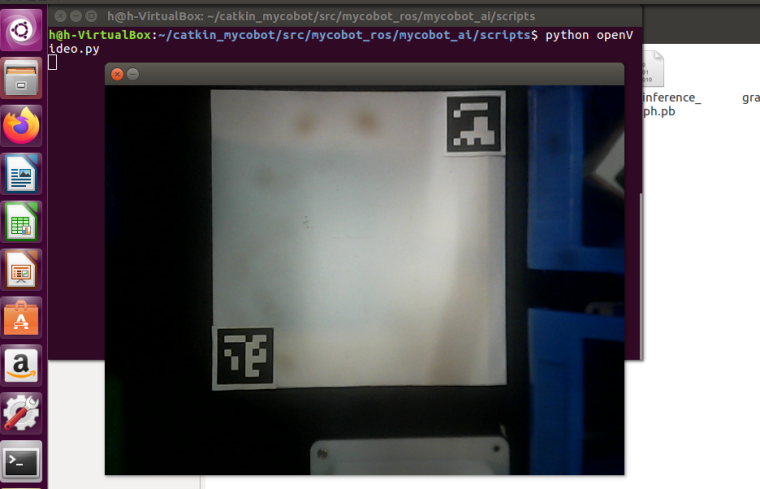# Implement the color recognition on myCobot

• MyCobot AI Kit is an entry-level artificial intelligence suit integrating vision, positioning and grabbing, and automatic sorting modules. Based on the Linux system, the 1:1 simulation model is built in ROS, and the control of the mechanical arm can be realized through the development of software. It is easy to use the training platform of colleges and universities, the construction of robotics disciplines, the robotics laboratory or personal learning and use.

With deep learning, users can use the color recognition function of the myCobot to complete location grabbing and automatic sorting.So how to implement the color recognition and control the myCobot to grab the modules? Let’s take a look.

First, we need to use Python to run openvideo. Py under the mycobot_ai package. Make sure that the camera completely cover the whole recognition area. This recognition area is square in the video, as shown in the figure below. If the recognition area does not meet the requirements in the video, the position of the camera needs to be adjusted.**2.Case demonstration

The operation process:**
Go to the mycobot_ai package in the mycobot-ros workspace through the file manager.

Right click to open the terminal.

Give permission to operate the manipulator, enter `sudo chmod 777 /dev/ttyU`

If the device name is not `/dev/ttyUSB0`, you need to change the port value in the vision. Launch file.

Enter `roslaunch launch/vision.launch` to open the vision. Launch file, which contains some core libraries and dependencies of ROS.

Create a marker in the rviz graphical interface and name it cube.

Type `ctrl+shift+t in` the command terminal to open another command window under the same directory.

Enter `Python script / detect_ obj_ Color. Py` open the color recognition program to realize color recognition and capture.

Note：
1)When the camera does not automatically frame the identification area correctly, it is necessary to close the program, adjust the position of the camera, and move the camera to the left or right side.

2)If the command terminal does not appear OK and the color cannot be recognized, the camera needs to be moved back or forward slightly. When the command terminal appears OK, the program can run normally.

3)OpenCV image recognition could be affected by the environment. If it is in a dark environment, the recognition effect will be greatly reduced.

The explaination of the code
This case is based on opencv and ROS communication control manipulator. First, calibrate the camera to ensure the accuracy of the camera. By identifying two aruco codes in the capture range, the recognition range is intelligently located, and the corresponding relationship between the center point of the actual recognition range and the video pixel is determined.

Use the color recognition function provided by opencv to identify the object block and determine the pixel position of the object block in the video, and calculate the coordinates of the object block relative to the center of the actual recognition range according to the pixel point of the object block in the video and the video pixel point of the center of the actual recognition range, Then, the relative coordinates of the object block relative to the manipulator can be calculated according to the relative coordinates between the center of the actual identification range and the manipulator. Finally, a series of actions are designed to grab the object block and place it in the corresponding bucket.

Still don't understand after reading? Don’t worry! Next, we will explain the whole implementation process step by step.

1)Identify aruco modules
Use the aruco recognition function of opencv to identify the aruco of the picture, and conduct some brief information filtering to obtain the pixel position information of two aruco.

``````    def get_calculate_params(self,img):
# Convert picture to gray picture
gray = cv2.cvtColor(img, cv2.COLOR_BGR2GRAY)
# Check whether there is aruco in the picture
corners, ids, rejectImaPoint = cv2.aruco.detectMarkers(
gray, self.aruco_dict, parameters=self.aruco_params
)

"""
It is required that there are two arucos in the picture in the same order.

There are two arucos in corners, and each aruco contains its four corner pixel bits.

The center position of aruco is determined according to the four corners of aruco.
"""
if len(corners) > 0:
if ids is not None:
if len(corners) <= 1 or ids==1:
return None
x1=x2=y1=y2 = 0
point_11,point_21,point_31,point_41 = corners
x1, y1 = int((point_11 + point_21 + point_31 + point_41) / 4.0), int((point_11 + point_21 + point_31 + point_41) / 4.0)
point_1,point_2,point_3,point_4 = corners
x2, y2 = int((point_1 + point_2 + point_3 + point_4) / 4.0), int((point_1 + point_2 + point_3 + point_4) / 4.0)
return x1,x2,y1,y2
return None
``````

2)Clip video module

According to the pixel points of two aruco, determine the pixel range of the recognition range in the video, and then cilpped.

``````   """
Expand the video pixel by 1.5x, that is, enlarge the video size by 1.5x.

If two *aruco* values have been calculated, video clipping is performed.
"""
def transform_frame(self, frame):
# Enlarge the picture 1.5x
fx = 1.5
fy = 1.5
frame = cv2.resize(frame, (0, 0), fx=fx, fy=fy, interpolation=cv2.INTER_CUBIC)
if self.x1 != self.x2:
# The clipping scale here is adjusted according to the actual situation
frame = frame[int(self.y2*0.4):int(self.y1*1.15), int(self.x1*0.7):int(self.x2*1.15)]
return frame
``````

3)Color recognition module
Chroma conversion is performed on the received picture, the picture is converted into gray picture, and the color recognition range is set according to HSV initialized by the user-defined class.

Corrode and expand the converted gray image to deepen the color contrast of the image. Identify and locate the color of the object block through filtering and checking the contour. Finally, through some necessary data filtering, color blocks are framed in the picture.

``````def color_detect(self, img):
x = y = 0
for mycolor, item in self.HSV.items():
redLower = np.array(item)
redUpper = np.array(item)
# Convert picture to gray picture
hsv = cv2.cvtColor(img, cv2.COLOR_BGR2HSV)
# Set color recognition range
# The purpose of etching the picture is to remove the edge roughness
erosion = cv2.erode(mask, np.ones((1, 1), np.uint8), iterations=2)
# Expand the picture to deepen the color depth in the picture
dilation =cv2.dilate(erosion, np.ones((1, 1), np.uint8), iterations=2)
# Add pixels to the picture
# Turn the filtered image into a binary image and put it in binary
ret, binary = cv2.threshold(dilation, 127, 255, cv2.THRESH_BINARY)
# Obtain the image contour coordinates, where contour is the coordinate value. Here, only the contour is detected
contours, hierarchy = cv2.findContours(
dilation, cv2.RETR_EXTERNAL, cv2.CHAIN_APPROX_SIMPLE)

if len(contours) > 0:
# Deal with the misidentification
boxes = [
box
for box in [cv2.boundingRect(c) for c in contours]
if min(img.shape, img.shape) / 10
< min(box, box)
< min(img.shape, img.shape) / 1
]
if boxes:
for box in boxes:
x, y, w, h = box
# Find the largest object that meets the requirements
c = max(contours, key=cv2.contourArea)
# Obtain the lower left and upper right points of the positioning object
x, y, w, h = cv2.boundingRect(c)
# Frame the block in the picture
cv2.rectangle(img, (x, y), (x+w, y+h), (153, 153, 0), 2)
# Calculate Block Center
x, y = (x*2+w)/2, (y*2+h)/2
# Judge what color the object is
if mycolor == "yellow":
self.color = 1
elif mycolor == "red":
self.color = 0

# Judge whether the identification is normal
if abs(x) + abs(y) > 0:
return x, y
else:
return None
``````

4. Grab the implementation module
​ A series of points are designed for the movement of the manipulator, such as the initialization point of the manipulator, the point to be grasped, the point above the blue bucket, the point above the green bucket, etc. In order to simulate the movement of the object block in rviz, a series of points are set for the movement of the object block. Since the model coordinates in rviz are in m and the manipulator coordinates are in mm, it is necessary to divide the data by 1000.

`````` def move(self, x,y,color):
angles = [
[-7.11, -6.94, -55.01, -24.16, 0, -38.84],  # Initialization point
[-1.14, -10.63, -87.8, 9.05, -3.07, -37.7],  # Point to be grabbed
[17.4, -10.1, -87.27, 5.8, -2.02, -37.7],  # Point to be grabbed
]

coords = [
[106.1, -141.6, 240.9, -173.34, -8.15, -83.11],  # Point above blue bucket
[208.2, -127.8, 246.9, -157.51, -17.5, -71.18],  # Point above green bucket
[209.7, -18.6, 230.4, -168.48, -9.86, -39.38],   # cube Point to be grabbed
[196.9, -64.7, 232.6, -166.66, -9.44, -52.47],   # cube Point to be grabbed
[126.6, -118.1, 305.0, -157.57, -13.72, -75.3],  # cube Point to be grabbed

]
# Send angle mobile manipulator
self.pub_angles(angles, 20)
time.sleep(1.5)
self.pub_angles(angles, 20)
time.sleep(1.5)
self.pub_angles(angles, 20)
time.sleep(1.5)
# Send coordinates to move the manipulator
self.pub_coords([x, y, 165,  -178.9, -1.57, -25.95], 20, 1)
time.sleep(1.5)
self.pub_coords([x, y, 110,  -178.9, -1.57, -25.95], 20, 1)
time.sleep(1.5)
# Start suction pump
self.pub_pump(True)
time.sleep(0.5)
self.pub_angles(angles, 20)
time.sleep(3)
self.pub_marker(coords/1000.0, coords/1000.0, coords/1000.0)

self.pub_angles(angles, 20)
time.sleep(1.5)
self.pub_marker(coords/1000.0, coords/1000.0, coords/1000.0)

self.pub_angles(angles, 20)
time.sleep(1.5)
self.pub_marker(coords/1000.0, coords/1000.0, coords/1000.0)

self.pub_coords(coords[color], 20, 1)
self.pub_marker(coords[color]/1000.0, coords[color]/1000.0, coords[color]/1000.0)
time.sleep(2)
# Turn off the suction pump
self.pub_pump(False)
if color==1:
self.pub_marker(coords[color]/1000.0+0.04, coords[color]/1000.0-0.02)
elif color==0:
self.pub_marker(coords[color]/1000.0+0.03, coords[color]/1000.0)
self.pub_angles(angles, 20)
time.sleep(3)
``````

5)Position calculation
By measuring the pixel positions of two aruco in the capture area, the pixel distance M1 between two aruco can be calculated, and the actual distance M2 between two aruco can be measured, so that we can obtain the ratio of pixels to actual distance ratio = m2 / M1.

We can calculate the pixel difference between the color object block and the center of the capture area from the picture, so we can calculate the relative coordinates (x1, Y1) of the actual distance of the object block from the center of the capture area.

Add the relative coordinates*(x1, Y1)* from the center of the gripping area to the manipulator (X2, Y2) to obtain the relative coordinates (X3, Y3) of the object block to the manipulator. The specific code implementation can view the program source code.

We also prepare a video demo for you:
Video Demo
Hope that this article can provide you some help! MyCobot AI Kit also owns the image and aruco code recognition, if you are interested in these programming learning, this kit is worthy of being considered!

• Good article, I will buy this kit